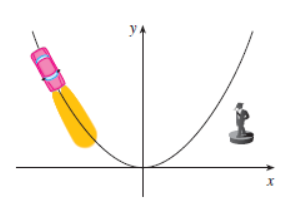# The point on the highway will the car’s headlights illuminate the status.### Single Variable Calculus: Concepts...

4th Edition
James Stewart
Publisher: Cengage Learning
ISBN: 9781337687805### Single Variable Calculus: Concepts...

4th Edition
James Stewart
Publisher: Cengage Learning
ISBN: 9781337687805

#### Solutions

Chapter 2, Problem 14P
To determine

## To find:The point on the highway will the car’s headlights illuminate the status.

Expert Solution

The point on the highway will the car’s headlights illuminate the status at (100502)m east and (1501002)m north to the origin.

### Explanation of Solution

Given information:

The car travelling along a highway shaped like parabola with its vertex at the origin as shown in figure (1).Figure (1)

Calculation:

The parabola has the vertex at (0,0) and has y -axis as its axis.it shows that the parabola is in the upper half plane and the equation of the parabola will be y=ax2 with a>0 .

The point (100,100) is on the parabola.

y=ax2100=(100)2a=1100

So, the equation of parabola is.

y=x2100

Assume that the headlight of the car illuminates the statue at the point of coordinate (x0,y0) .

y0=x02100

The coordinate of the statue is (100,50) , the tangent line to the parabola at point (x0,y0) passes through the point (100,50) .

Calculate the slope of the tangent line at any point is.

y'=2x100=x50

Theslope of the tangent line at (x0,y0) is.

y'=x050

The equation of tangent line is.

(yy0)=x050(xx0)

It passes through the point (100,50) .

(50y0)=x050(100x0)50y0=2x0x0250

As we know that, y0=x02100 .

50y0=2x02y0y0=2x050

Substitute x02100 for y0 above linear equation.

x02100=2x050x02200x0+5000=0x0=100±502

The minus sign is the right one, and the other one corresponds to when the taillights of the car would be illuminating the statue.

The value of y0 will be.

y0=2(100502)50=200100250=150100250

Therefore, the point on the highway will the car’s headlights illuminate the status at (100502)m east and (1501002)m north to the origin.

### Have a homework question?

Subscribe to bartleby learn! Ask subject matter experts 30 homework questions each month. Plus, you’ll have access to millions of step-by-step textbook answers!